# Topic:

As a pet lover, the challenge from Kaggle about recognizing whether the animal in the picture is a cat or a dog has caught my attention. The “Dogs vs Cats” challenge provides a dataset about pictures of cats and dogs. This image recognition problem then asks participants to build an algorithm to classify whether pictures from the uncategorized test data contain either a dog or a cat. Although this challenge is easy to be done with human eyes, it is hard for computers to classify. In this report, I will explore the data from Kaggle, train and compare multiple ML models, and articulate why I choose my final model at the end.

# Dataset:

I extracted the dogs-vs-cats dataset from Kaggle, with 2 zip files: “train.zip” and “test1.zip”.

The “train.zip” refers to training data, which contains the pictures of dogs/cats, picture ids, and labels — either a dog or a cat is in the picture. For example, a cat picture in the training data may have the name “cat.1024.jpg”, as shown below.

The “test1.zip” refers to testing data, which contains the pictures of dogs/cats, picture ids, but not labels, and is used to test models and submit to Kaggle challenge. For example, a cat picture in the testing dataset may have the name “1024.jpg”, as shown below.

# Data Collection

Here are a few random dog photos from the dogs-vs-cat dataset:

And here are a few random cat photos from the dogs-vs-cat dataset:

In the code, dogs are encoded as 1, and cats are encoded as 0. Here’s an example of the data frame:

All entries in the data frame are non-null, thus there’s no need to further clean the null data.

From the 25000 entries of data in the training dataset, we have 125000 cats and 125000 dogs, indicated in the picture below.

We select 25% of the pictures (about 25000 * 0.25 = 6250 pictures) to be used for validation (as the test dataset for our model). Because the sizes of the pictures are different in the original data, we create an instance of the ImageDataGenerator that will rescale the pixel values of the pictures which further be fitted into the models discussed below.

# Models

## Model 1: Neural Network Model with One Convolution Layer

There is first a convolution layer — the combination of Conv2D and MaxPool2D layers, with Rectified Linear Unit (relu) as the activation function. Then we flatten the array and create a hidden Dense layer with 128 units and use Rectified Linear Unit (relu) as the activation function. The very last part is the output layer, which contains a value of 0 or 1, with 0 representing a cat and 1 representing a dog. The learning rate of the optimizer is 0.001.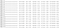History of training accuracy and loss in each epoch (Model 1)

Evaluating the model, we have a loss of 0.6886 and an accuracy of 0.5369. From the pictures below for the differences of accuracy and loss between training and validation sets. In the first picture, training accuracy and validation accuracy are increasing together to about 0.66 at the 3rd epoch. And after that, validation accuracy is relatively low compared to training accuracy. In the second picture, training loss and validation loss are decreasing together to about 0.61 at the 3rd epoch. After 3rd epoch, training loss is relatively lower than validation loss.

Thus, it seems that, from the picture, Model 1 has overfitted the training dataset at about the 3rd epoch.

## Model 2: Neural Network Model with Two Convolution Layers

The model with two convolution layers extends Model 1 by adding one additional convolution layer with 64 filters.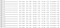History of training accuracy and loss in each epoch (Model 2)

Evaluating the model, we have a testing loss of 0.5528 and a testing accuracy of 0.7189. From the pictures below for the differences of accuracy and loss between training and validation sets. In the first picture, training accuracy and validation accuracy are increasing together to about 0.71–0.73 at the last epoch. In the second picture, training loss and validation loss are decreasing together to about 0.53–0.55 at the last epoch.

## Model 3: Neural Network Model with Three Convolution Layers

This model with three convolution layers extends Model 2 by adding one additional convolution layer with 128 filters.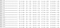History of training accuracy and loss in each epoch (Model 3)

Evaluating the model, we have a testing loss of 0.5655 and a testing accuracy of 0.7076. From the pictures below for the differences of accuracy and loss between training and validation sets. In the first picture, training accuracy and validation accuracy are increasing together to about 0.70 at the last epoch. In the second picture, training loss and validation loss are decreasing together to about 0.55 at the last epoch.

# Simplifying the Model (Dropout Regularization)

Since from the picture in Model 1, we can tell that there is an overfit for the training dataset after the 3rd epoch. Thus, to simplify the model and reduce overfitting, we add dropout regularization to Model 1.

The simplified model extends Model 1 by adding the dropout regularization. In this model, a 20% dropout rate is applied after the convolution layer, and a 50% dropout rate applied after the fully connected layer in the classifier part of the model.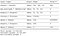Neural Network Model with One Convolution Layer (with Dropout Regularization)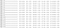History of training accuracy and loss in each epoch (Model 1 with Dropout Regularization)

Evaluating the model, we have a testing loss of 0.7107 and a testing accuracy of 0.5146. From the pictures below for the differences of accuracy and loss between training and validation sets. In the first picture, training accuracy and validation accuracy are increasing together to about 0.66 at the last epoch. In the second picture, training loss and validation loss are decreasing together to about 0.61 at the last epoch.

Graph for Model 1 without the dropout regularization is shown below, which involves overfitting after epoch 3.

Thus, by comparing the performance of Model 1 with and without dropout regularization, we can tell that the overall accuracy does not improve much, but the overfitting has been reduced or delayed.

# Discussion

For the three models with different amounts of convolution layers (the combination of Conv2D and MaxPool2D layers), the accuracies of the models are as follows:

• Model 1 has an accuracy of 0.5369 (one convolution layer).
• Model 2 with an accuracy of 0.7189 (two convolution layers).
• Model 3 has an accuracy of 0.7076 (three convolution layers).
• Model 1 with dropout regularization has an accuracy of 0.5164 (one convolution layer).

From the results above, we can tell that there’s an increase in accuracies between one and two convolution layer(s) but not in accuracies between two and three convolution layers. Thus, there’s no overall pattern between the number of layers and the accuracy of the model.

Besides, by adding dropout regularization to Model 1, the accuracy is not increased much. However, the obvious overfitting behavior after epoch 3 for Model 1 has been reduced or delayed to a great extent. For further exploration and optimization, a larger dropout rate after the convolution layer could be considered.

In order to make the model most accurate, I choose Model 3 as my final model. For further overfitting problems, dropout regularization or image augmentation could be used.

# Github Repo:

https://github.com/Yinghui-HE/Dogs-vs-Cats